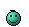News: Visit the official fractalforums.com Youtube Channel## The All New FractalForums is now in Public Beta Testing! Visit FractalForums.org and check it out!

ColourShockPrevious Image | Next Image
Description: Tweak of Theli-at's Pseudo Kleinian (PseudoKleinian-Progressive-DOF.frag) in the Experimental Examples in Fragmentarium, but with DE-Kn2cr11-raytracer.
Trying to get to grips with colours and light.
2000 subframes, and with 30% of padding still had to edit the whole image to remove the tile edges :} It's going to be 1-tile renders from now onCode:
#info Notice: set Render mode to Continous
#infoTheli-at's Pseudo Kleinian (Scale 1 JuliaBox + Something
#include "DE-Kn2cr11.frag"
#include "MathUtils.frag"
#group PseudoKleinian

#define USE_INF_NORM

// http://www.fractalforums.com/3d-fractal-generation/fragmentarium-an-ide-for-exploring-3d-fractals-and-other-systems-on-the-gpu/msg32270/#msg32270

// Maximum iterations
uniform int MI; slider[0,5,60]

// Bailout
//uniform float Bailout; slider[0,20,1000]

// Size
uniform float Size; slider[0,1,2]

// Cubic fold Size
uniform vec3 CSize; slider[(0,0,0),(1,1,1),(2,2,2)]

// Julia constant
uniform vec3 C; slider[(-2,-2,-2),(0,0,0),(2,2,2)]

// Thingy thickness
uniform float TThickness; slider[0,0.01,2]

// Thingy DE Offset
uniform float DEoffset; slider[0,0,0.01]

// Thingy Translation
uniform vec3 Offset; slider[(-1,-1,-1),(0,0,0),(1,1,1)]

float RoundBox(vec3 p, vec3 csize, float offset)
{
vec3 di = abs(p) - csize;
float k=max(di.x,max(di.y,di.z));
return abs(k*float(k<0.)+ length(max(di,0.0))-offset);
}

float Thingy(vec3 p, float e){
p-=Offset;
return (abs(length(p.xy)*p.z)-e) / sqrt(dot(p,p)+abs(e));
}

float Thing2(vec3 p){
//Just scale=1 Julia box
float DEfactor=1.;
vec3 ap=p+1.;
for(int i=0;i<MI && ap!=p;i++){
ap=p;
p=2.*clamp(p, -CSize, CSize)-p;

float r2=dot(p,p);
orbitTrap = min(orbitTrap, abs(vec4(p,r2)));
float k=max(Size/r2,1.);

p*=k;DEfactor*=k;

p+=C;
orbitTrap = min(orbitTrap, abs(vec4(p,dot(p,p))));
}
//Call basic shape and scale its DE
//return abs(0.5*Thingy(p,TThickness)/DEfactor-DEoffset);

//Alternative shape
//return abs(0.5*RoundBox(p, vec3(1.,1.,1.), 1.0)/DEfactor-DEoffset);
//Just a plane
return abs(0.5*abs(p.z-Offset.z)/DEfactor-DEoffset);
}

float DE(vec3 p){
return  Thing2(p);//RoundBox(p, CSize, Offset);
}

#preset Default
FOV = 1.165636
Eye = -0.153698,2.985096,2.326648
Target = 0.6932328,-1.231008,-6.397727
Up = 0.1447118,0.8945708,-0.4182583
Gamma = 0.8462079
ToneMapping = 5
Exposure = 0.9356436
Brightness = 1
Contrast = 1
Saturation = 1
GaussianWeight = 1
AntiAliasScale = 2
Detail = -3.377451
FudgeFactor = 1
Dither = 0.0370629
NormalBackStep = 1
CamLight = 0.3490196,0.3490196,0.3490196,0.0814868
BaseColor = 1,0.9882353,0.9490196
OrbitStrength = 0.9765791
X = 0.7568627,0.9098039,0.9098039,0.6887648
Y = 1,0.9803922,0.8980392,0.6692254
Z = 0.7843137,0,1,0.5973417
R = 1,0.7019608,0,1
BackgroundColor = 0,0.333333,1
CycleColors = true
Cycles = 17.11808
EnableFloor = false
FloorNormal = 0,0,0
FloorHeight = 0
FloorColor = 1,1,1
UpLock = false
FocalPlane = 0.3665621
Aperture = 0.0112994
InFocusAWidth = 0.9386724
DofCorrect = true
ApertureNbrSides = 7
ApertureRot = 0
ApStarShaped = false
Bloom = true
BloomIntensity = 0.5778098
BloomPow = 2
BloomTaps = 21
BloomStrong = 3.509691
RefineSteps = 4
MaxRaySteps = 429
MaxDistance = 10
DetailAO = -0.5
coneApertureAO = 0.5
maxIterAO = 20
FudgeAO = 1
AO_ambient = 1.144068
AO_camlight = 1.755477
AO_pointlight = 1.903546
AoCorrect = 1
Specular = 0.4
SpecularExp = 16
AmbiantLight = 1,0.9529412,0.8666667,1.381608
Reflection = 0.9882353,0.01568627,0.01568627
ReflectionsNumber = 1
SpotGlow = true
SpotLight = 0.4352941,0.4352941,0.4352941,0.6104651
LightPos = -5.557133,0,0
LightSize = 0.0783345
LightFallOff = 0.0156695
LightGlowExp = 1.09319
perf = false
SSS = false
sss1 = 0.1
sss2 = 0.5
HF_Fallof = 0.1
HF_Const = 0.1064426
HF_Intensity = 0.113942
HF_Dir = 0,0,1
HF_Offset = 2.734982
HF_Color = 0.5411765,0.8470588,0.8745098,0.8899214
HF_Scatter = 0
HF_Anisotropy = 0,0,0
HF_FogIter = 1
EnCloudsDir = false
Clouds_Dir = 0,0,1
CloudScale = 1
CloudFlatness = 0
CloudTops = 1
CloudBase = -1
CloudDensity = 1
CloudRoughness = 1
CloudContrast = 1
CloudColor = 0.65,0.68,0.7
CloudColor2 = 0.07,0.17,0.24
SunLightColor = 0.7,0.5,0.3
Cloudvar1 = 0.99
Cloudvar2 = 1
CloudIter = 5
CloudBgMix = 1
MI = 60
Size = 1.15094
CSize = 0.92436,1.21212,1.0101
C = -0.1530055,-0.057377,-0.03508
TThickness = 0.01
DEoffset = 0
Offset = 0.1638889,0.0416667,-0.3569444
#endpreset

Stats:
Total Favorities: 0 View Who Favorited
Filesize: 3.97MB
Height: 1074 Width: 1914
Discussion Topic: View Topic
Keywords: Fragmentarium Theli-at progressive dof-tweak
Posted by: SabineSeptember 26, 2016, 11:49:16 PM

Rating:by 1 members.